# (26) The action of some commercial drain cleaners is based on the following reaction:2 NAOH(s) + 2 Al(s) + 6 H2O(1) → 2 NaAl(OH)4(s) + 3 H2(g)What is the volume of H2 gas formed at STP when 6.32 g of Al reacts with excess NaOH?A) 3.50 LB) 5.25 LC) 7.87 LD) 8.59 L

Question
166 views

I'm not sure how to do this one.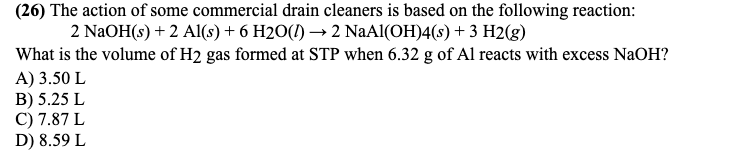help_outlineImage Transcriptionclose(26) The action of some commercial drain cleaners is based on the following reaction: 2 NAOH(s) + 2 Al(s) + 6 H2O(1) → 2 NaAl(OH)4(s) + 3 H2(g) What is the volume of H2 gas formed at STP when 6.32 g of Al reacts with excess NaOH? A) 3.50 L B) 5.25 L C) 7.87 L D) 8.59 L fullscreen
check_circle

Given data: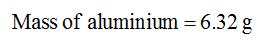Calculations:

The molar mass of aluminium (Al) is 26.98 g/mol.

The number of moles of aluminium is calculated as shown below.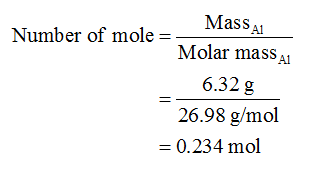The given reaction is shown below.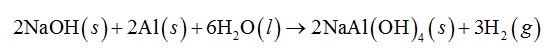In the above reaction, 2.0 mol of aluminium (Al) produces 3.0 mol of hydrogen gas (H2). Therefore, the number of moles of hydrogen gas produced by 0.234 mol of aluminium (Al) is calculated as shown below.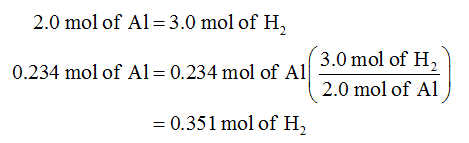At STP, the pressure (P) is 1.0 atm and temperature (T) is 273.15 K. The volume of the hydrogen gas is calculated by the formula shown below.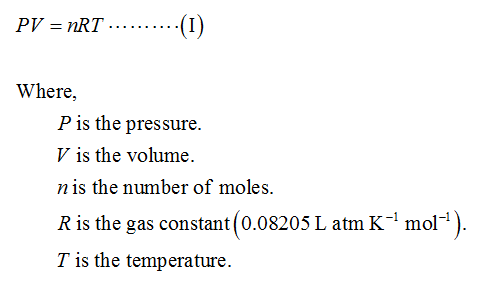Substitute the given data in above equation (I).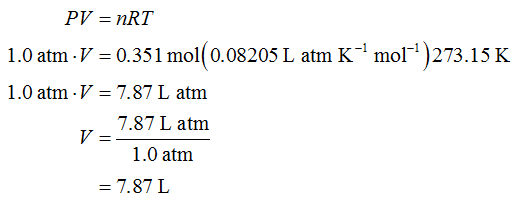...

### Want to see the full answer?

See Solution

#### Want to see this answer and more?

Solutions are written by subject experts who are available 24/7. Questions are typically answered within 1 hour.*

See Solution
*Response times may vary by subject and question.
Tagged in